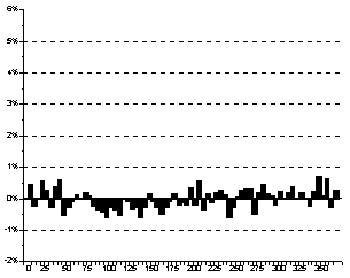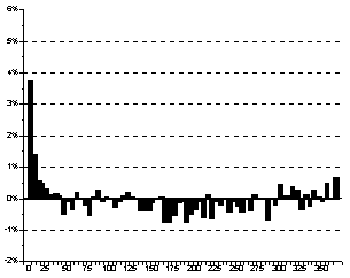The Manifesto | The Dominion | Texts and Articles | Review | Links | ACCUEIL (FR) | HOME (EN)

 Questioning Methods by Didier Castille

Abstract: Two statistical examinations conducted on very different populations and using the same methodology lead to rather similar results. A doubt emerges from both these facts : we are maybe facing an artefact. In this article, different methodology steps are criticized one by one but do not permit to identify the artefact we suspected. The conclusions drawn from each of the two studies remain unexplained.

Two experiments, one method, similar results

We observed 6 498 320 marriages which were registered by the French registry offices between 1976 and 1997 (see Sunny Day for a Wedding ), and 10 081 752 deceases of people over one which were registered in France between 1979 and 1997 by the same registry offices (see A Link between Birth and Death ).

Both these studies were based on the same null hypothesis, meaning that two dates are independent one another :

• for the first study, the wifes birth date is independent of the husbands date of birth,
• for the second study, the date of decease is independent of the deceased person birth date.
The same methodology was performed for both studies, in order to test the null hypothesis validity :
• in a first step, the Suns longitude at 12 noon G.M.T. was attributed to each date ; the solar longitudes were arranged into twelve classes (astrological signs),
• next, the observations were distributed into a 144-cell table (12 signs for each date),
• then, a theoretical distribution was calculated, compared to the actual distribution and a Chi-square test was performed,
• for each study, the Chi-square value was quite high and the null hypothesis was rejected with a very slight error risk,
• the analysis of the Chi-square contributions showed that, for each study, the combinations of same solar signs were significantly more numerous than predicted.
Consequently, we were very doubtful in front of these unexpected results. It was imperative to perform controls in order to test the coherence of the statistics. Three points of view were considered :
• the first one questioned the actual distribution. The number of people we used was extremely vast and the phenomena we observed, though they were significant, were rather slight. Maybe these results were ineluctable with such vast populations. The control we imagined consisted in performing the tests on random combinations of signs.
• the second one questioned the way we attributed a solar longitude to each date. The controls we imagined consisted in using an ordinary calendar. In a first step, we tested the independence of month of birth and month of decease, taking the number of days of each month into account. In a second step, as far as the birthday period seemed to be linked with the date of decease, we calculated the number of days separating the two dates on both actual population and random population.
• the third one questioned the sturdiness of the statistical tool we used, in other words the Chi-square test, as far as we were working on vast populations. Here, we tried to know empirically whether the sample size was the principal factor that could produce a high Chi-square value and, consequently, prove the significance of the results.

First control : working on fictitious populations

The following tables schematize, for each combinations of signs; the deviation of the expected values from the actual values for the weddings and for the deceases. The left-hand tables represent the effective populations, the right-hand tables represent the random populations. These fictitious populations were built by dividing the original files in two parts (one containing the wives and the other containing the husbands ; one containing the dates of birth and the other containing the dates of decease) and by creating randoms combinations. Our objective was to compare the actual populations and the fictitious populations.

Weddings

 Breakdown of actual couples Breakdown of random couples
 ♈ ♉ ♊ ♋ ♌ ♍ ♎ ♏ ♐ ♑ ♒ ♓ ♈ ♉ ♊ ♋ ♌ ♍ ♎ ♏ ♐ ♑ ♒ ♓ ♈ * Aries * ♈ ♉ * * * * * * * Taurus ♉ ♊ * * * * * Gemini ♊ ♋ * * * Cancer ♋ ♌ * * * Leo * ♌ ♍ * * * * * Virgo ♍ ♎ * * * Libra ♎ ♏ * * * Scorpio ♏ ♐ * * * * Sagittarius ♐ ♑ * * * * Capricorn ♑ ♒ * * * * Aquarius ♒ ♓ * * * * Pisces * * * ♓

NB : the columns of the tables correspond to the solar sign of the wife, the rows correspond to solar sign of the husband.

The colored cells highlight those combinations for which the actual number is greater than the theoretical number. The amplitude indicators of the contribution (the asterisks) can be translated as follows :

 the indicator is : It means that the deviation is : * * * significant with a risk of 0.5% at the most * * significant with a risk of 1% at the most * significant with a risk of 5% at the most not significant

Deceases

 Breakdown of actual deceases Breakdown of random deceases
 ♈ ♉ ♊ ♋ ♌ ♍ ♎ ♏ ♐ ♑ ♒ ♓ ♈ ♉ ♊ ♋ ♌ ♍ ♎ ♏ ♐ ♑ ♒ ♓ ♈ Aries ♈ ♉ * * * Taurus ♉ ♊ Gemini * ♊ ♋ * * * * * Cancer * * ♋ ♌ * * * Leo ♌ ♍ Virgo * * ♍ ♎ * Libra * ♎ ♏ * * Scorpio * ♏ ♐ * * * * Sagittarius * ♐ ♑ * * * * Capricorn ♑ ♒ * * * * Aquarius * ♒ ♓ * * * Pisces ♓

NB : the columns of the tables correspond to the solar sign at decease time, the rows correspond to solar sign at birth.

The differences between left-hand tables and right-hand tables are obvious : the Chi-square values are not only very different (295.1 and 119.4 for the weddings ; 229.7 and 147.7 for the deceases) but the diagonals of significant excess observations are neat in the left-hand tables but absent in the right-hand tables.

Second control : the time reference

First, we tested the independence of the month of birth and the month of decease on the entire population. The Chi-square value reached 271.97, meaning that the null hypothesis could be rejected with a very slight risk of error.

Secondly, we took into account the number of days composing the months by weighting each observation. For example, for someone who was born in May (31 days) and who died in June (30 days), we used a weighting of 0.968 (=[30/31]*[30/30]). Weighted in this fashion, the population contained 9 801 490 deceases. The test we performed on this weighted population produced a Chi-square value of 265.42 ; this value confirmed the previous result.

Next, we calculated the number of days separating the day of birth and the day of decease. Then, we distributed the observations according to 73 classes of 5 days each and we compared the frequency of each class to the mean frequency (see left-hand histogram). The same operations were performed on the fictitious population (right-hand histogram).

 Breakdown of actual deceases Breakdown of random deceasesIn short, the null hypothesis can be rejected, wether we use a zodiacal reference or a ordinary calendar reference to locate the observations in time.

Third control : the impact of the sample size

Have the very large size of the populations we used an influence on the reliability of the Chi-square statistic? This statistic reflects the sum of all the squared relative deviations of actual values from theoretical values. Consequently, a mere deviation of 700 observations out of 70 000 expected produces a contribution of 7 : that is high enough for being considered as significant.

In other words, with such vast populations, maybe we can always expect an elevated Chi-square value as soon as some slight deviations exist.

The following example shows that it is not true. We tested the independence of the day of birth in the week (Sunday, Monday, etc.) and the day of decease in the week. We obtained a weak Chi-square value (20.9), meaning that we can reject the null hypothesis with an enormous error risk (97.9%). None of the contributions is significant.

Deviation of the actual values from the theoretical value according to the day of birth and to the day of decease

 Sunday Monday Tuesday Wednesday Thursday Friday Saturday Sunday Monday Tuesday Wednesday Thursday Friday Saturday

NB : the columns of the table correspond to the day of decease, the rows correspond to the day of birth.

This control proves that a Chi-square test performed on vast populations is not systematically significant.

Conclusion

Weddings and deceases registered by the French registry offices show statistical links that highlight the birthday period. We suspected an artefact related to the method we used. From the controls we performed, the building of the theoretical distributions, the time reference and the statisticals tests can not be questioned. An explanation remains to be found.